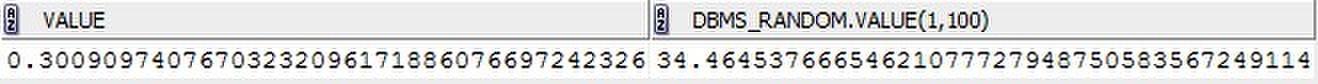# Oracle取随机数函数

The basic function gets a random number, greater than or equal to 0 and less than 1, with 38 digits to the right of the decimal (38-digit precision).
Alternatively, you can get a random Oracle number x, where x is greater than or equal to low and less than high.

SELECT DBMS_RANDOM.VALUE,DBMS_RANDOM.VALUE(1,100) FROM DUAL;• 博文量
102
• 访问量
904498